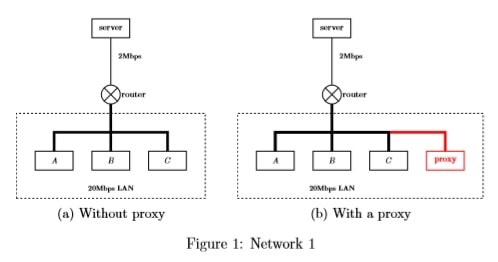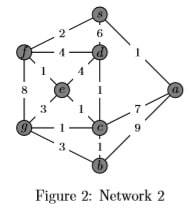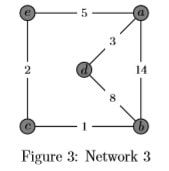# SEEM5770/ECLT5840 open systems/electronic commerce

```{`The Chinese University of Hong Kong
Department of Systems Engineering and Engineering Management
`}```

## Assignment 1: Networking

Q-1: Consider the following scenario which is demonstrated in Figure 1a: Users A, B and C are in an institutional network with 20Mbps LAN. A server that holds two web pages, namely, “homework.html” and “project.html”, is connected to the institutional network with a 2Mbps link. The “homework.html” and “project.html” are both of size 1M bits. Specially, “homework.html” references 3 figures, each of which is 0.3M bits. The server supports only non-persistent HTTP. To download an object from the server, it needs totally 20,000 bits (including requests and responses) to create a TCP connection. A HTTP request is 10,000 bits, and a HTTP response is 10,000 bits plus the size of the object contained. Processing delay, queuing delay, and propagation delay are assumed to be 0 in this question.

• What is the total time for user A to download “homework.html” and then “project.html”?
• If a proxy server is installed for the institutional network (see Figure 1b), what is the total time for user A to download “homework.html” and then “project.html”? Compare with (a).
• After A has finished, how much time it takes for B to download “homework.html” and then for C to download “project.html” provided the proxy server is present, comparing with the question (b)?Q-2: Given all the fields but the checksum field of a UDP segment shown in Table 1, calculate the checksum field. The structure of Table 1 is as follows: the first two lines are the header of the segment, while the remaining two lines are payloads in the segment.

 0000 0000 0000 0101 0000 0000 0010 0011 0000 0000 0010 0000 checksum 1000 1001 0101 0011 0111 1111 0011 0000 1010 1101 0100 0111 1111 0101 0000 1110

Table 1: A UDP segment with checksum unknown

Q-3: Consider a reliable data transfer protocol that uses only negative acknowledgments (NAK). Suppose the sender sends data only infrequently. Would a NAK-only protocol be preferable to a protocol that uses ACKs? Why?Q-4: Consider the network shown in Figure 2. With the indicated link costs, use Dijkstra’s shortest-path algorithm to compute the shortest path from s to all network nodes. Show how the algorithm works by computing a table similar to the table shown in the lecture note of Chapter45th.ppt slide 4-21. And show the forwarding table for the router represented by s.Q-5: Consider the network shown in Figure 3. Assume that each node initially knows the costs to each of its neighbors. Following the distance-vector algorithm, show the steps to compute the distance tables until it is converged.